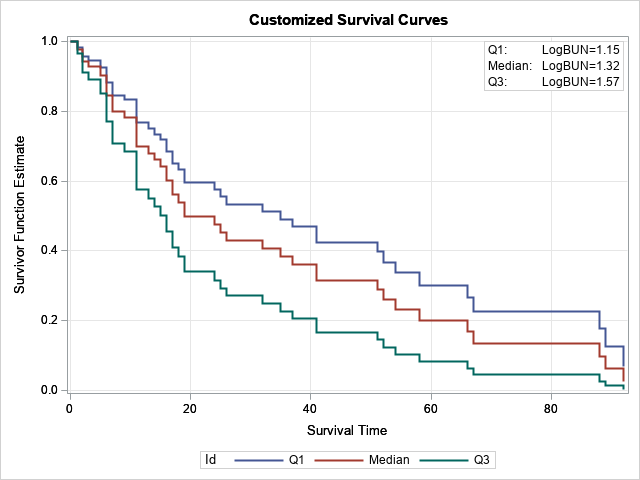This article shows how to create a "sliced survival plot" for proportional-hazards models that are created by using PROC PHREG in SAS. Graphing the result of a statistical regression model is a valuable way to communicate the predictions of the model. Many SAS procedures use ODS graphics to produce graphs automatically or upon request. The default graphics from SAS procedures are sufficient for most tasks. However, occasionally I customize or modify the graphs that are produced by SAS procedures.

The graph to the right is an example of how to create a customized sliced fit plot from PROC PHREG. This article shows how to create the plot and others like it.

It is worth mentioning that SAS supports multiple procedures for analyzing survival data. Of these, PROC LIFETEST has the most extensive methods for customizing graphics. An entire chapter of the SAS/STAT Users Guide is devoted to customizing the Kaplan-Meier plot, which is a popular graph in the life sciences.

### Sliced fit plots in SAS regression procedures

Before discussing PROC PHREG, let's review the concepts for a sliced fit plot for a regression model. In a "sliced fit plot," you display the predicted values for the model (typically versus a continuous covariate) for a specific value of the other continuous covariates. Often the mean value is used for slicing, but other popular choices include the quartiles: the 25th percentile, the median, and the 75th percentile.

My favorite way to create fit plots is to use the EFFECTPLOT SLICEFIT statement in PROC PLM. By default, the EFFECTPLOT statement evaluates the continuous covariates at their mean values, but you can use the SLICEBY= option or the AT= option to specify other values.

PROC PLM and the EFFECTPLOT statement were designed primarily for generalized linear models. If you try to use the EFFECTPLOT statement for other kinds of regression models (or nonparametric regressions), you might get a warning: WARNING: The EFFECTPLOT statement cannot be used for the specified model. In that case, you can manually create a sliced fit plot by scoring the model on a carefully prepared data set.

### Example data and the default behavior of PROC PHREG

PROC PHREG can create graphs automatically, so let's start by looking at the default survival plot. The following data is from an example in the PROC PHREG documentation. The goal is to predict the survival time (TIME and VSTATUS) of patients with multiple myeloma based on the measured values (log-transformed) of blood urea nitrogen (BUN). The example in the documentation analyzes nine variables, but for clarity, I only include two explanatory variables in the following data and examples:

```data Myeloma; input Time VStatus LogBUN Frac @@; label Time='Survival Time' VStatus='0=Alive 1=Dead'; datalines; 1.25 1 2.2175 1 1.25 1 1.9395 1 2.00 1 1.5185 1 2.00 1 1.7482 1 2.00 1 1.3010 1 3.00 1 1.5441 0 5.00 1 2.2355 1 5.00 1 1.6812 0 6.00 1 1.3617 0 6.00 1 2.1139 1 6.00 1 1.1139 1 6.00 1 1.4150 1 7.00 1 1.9777 1 7.00 1 1.0414 1 7.00 1 1.1761 1 9.00 1 1.7243 1 11.00 1 1.1139 1 11.00 1 1.2304 1 11.00 1 1.3010 1 11.00 1 1.5682 0 11.00 1 1.0792 1 13.00 1 0.7782 1 14.00 1 1.3979 1 15.00 1 1.6021 1 16.00 1 1.3424 1 16.00 1 1.3222 1 17.00 1 1.2304 1 17.00 1 1.5911 0 18.00 1 1.4472 0 19.00 1 1.0792 1 19.00 1 1.2553 1 24.00 1 1.3010 1 25.00 1 1.0000 1 26.00 1 1.2304 1 32.00 1 1.3222 1 35.00 1 1.1139 1 37.00 1 1.6021 0 41.00 1 1.0000 1 41.00 1 1.1461 1 51.00 1 1.5682 1 52.00 1 1.0000 1 54.00 1 1.2553 1 58.00 1 1.2041 1 66.00 1 1.4472 1 67.00 1 1.3222 1 88.00 1 1.1761 0 89.00 1 1.3222 1 92.00 1 1.4314 1 4.00 0 1.9542 0 4.00 0 1.9243 0 7.00 0 1.1139 1 7.00 0 1.5315 0 8.00 0 1.0792 1 12.00 0 1.1461 0 11.00 0 1.6128 1 12.00 0 1.3979 1 13.00 0 1.6628 0 16.00 0 1.1461 0 19.00 0 1.3222 1 19.00 0 1.3222 1 28.00 0 1.2304 1 41.00 0 1.7559 1 53.00 0 1.1139 1 57.00 0 1.2553 0 77.00 0 1.0792 0 ;```

Suppose you want to predict survival time by using LogBUN as the only explanatory variable. The following call to PROC PHREG builds the model and creates a survival plot:

```/* A COVARIATES= data set is not specified, so the average value for LogBUN is used in the survival plot */ proc phreg data=Myeloma plots(overlay)=survival; /* use plots(overlay CL) to get confidence limits */ model Time*VStatus(0)=LogBUN; run;```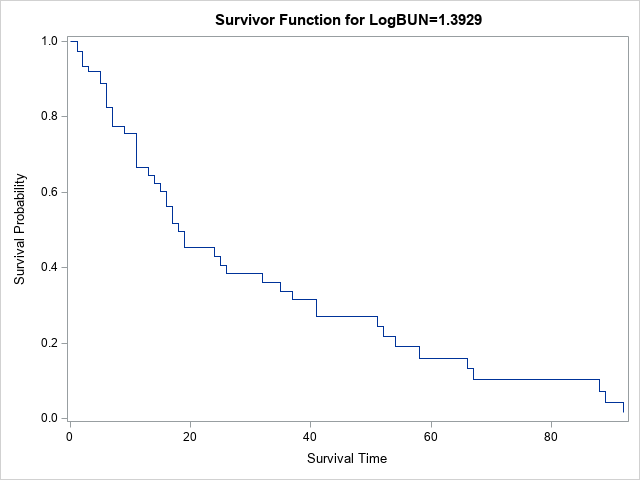Notice the title of the plot specifies that the survivor function is evaluated for LogBUN=1.3929. Why this strange value? Because this program did not use the BASELINE statement to specify a COVARIATES= data set. Consequently, the average value for LogBUN is used in the survival plot. You can use PROC MEANS to confirm the mean value of the LogBUN variable and to output other statistics such as the sample quartiles:

```proc means data=Myeloma Q1 Median Mean Q3; var LogBUN; run;```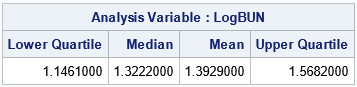The next section shows how to specify the quartiles as slicing values for LogBUN when you create a survival plot.

### Specify slicing values for a survival plot

PROC PHREG has a special syntax for producing a sliced fit plot. On the BASELINE statement, use the COVARIATES= option to name a SAS data set in which you have specified values at which to slice the covariates. If you do not specify a data set, or if your data set does not list some of the variables in the model, then the model is evaluated at the mean values for the continuous variables and at the reference levels for the CLASS variables.

Suppose you want to use the PHREG model to compare the probability of survival for three hypothetical patients whose LogBUN values are 1.15, 1.32, and 1.57, respectively. These LogBUN values for these patients are the sample quartiles for the distribution of patients in the study. The following DATA step creates a SAS data set that contains the quartile values. If you add the BASELINE statement and the COVARIATES= option, then PROC PHREG automatically incorporates those values into the survival graph that it creates:

```data InRisks; length Id \$9; input Id LogBUN; datalines; Q1 1.15 Median 1.32 Q3 1.57 ;   proc phreg data=Myeloma plots(overlay)=survival; /* use plots(overlay CL) to get confidence limits */ model Time*VStatus(0)=LogBUN; baseline covariates=Inrisks / rowid=Id; run;```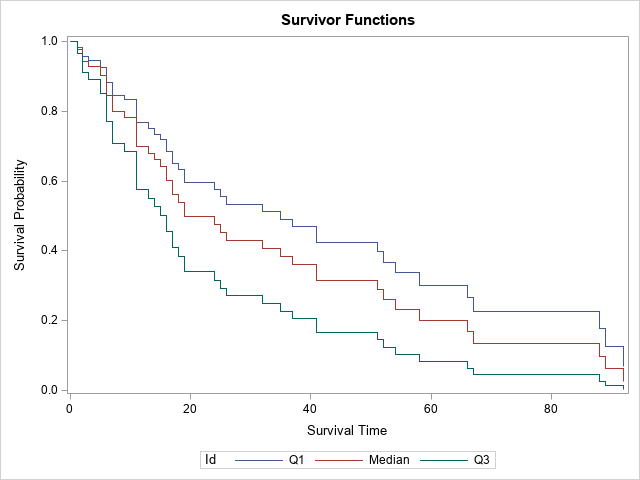The survival graph now displays three curves, one for each specified value of the LogBUN variable. The curves are labeled by using the ROWID= option on the BASELINE statement. If you omit the option, the values of the LogBUN variable are displayed.

### Create your own custom survival plot

This article was motivated by a SAS programmer who wanted to customize the survival plot. For simplicity, let's suppose that you want to modify the title, add a grid, increase the width of the lines, and add an inset. In that case, you can use the OUT= option to write the relevant predicted values to a SAS data set. The SURVIVAL= option specifies the name for the variable that contains the survival probabilities. However, if you use the keyword _ALL_, then the procedure outputs estimates, standard errors, and confidence limits, and provides default names. For the _ALL_ keyword, "Survival" is used for the name of the variable that contains the probability estimates. You can then use PROC SGPLOT to create a custom survival plot, as follows:

```/* write output to a SAS data set */ proc phreg data=Myeloma noprint; model Time*VStatus(0)=LogBUN; baseline covariates=Inrisks out=PHOut survival=_all_ / rowid=Id; run;   title "Customized Survival Curves"; proc sgplot data=PHOut; *band x=Time lower=LowerSurvival upper=UpperSurvival / group=Id transparency=0.75; step x=Time y=Survival / group=Id lineattrs=(thickness=2); inset ("Q1:"="LogBUN=1.15" "Median:"="LogBUN=1.32" "Q3:"="LogBUN=1.57") / border opaque; xaxis grid; yaxis grid; run;```

### Including a classification variable

The Myeloma data contains a categorical variable (Frac) that indicates whether the patient had bone fractures at the time of diagnosis. If you want to include that variable in the analysis, you can do so. You can add that variable to the COVARIATES= data set to control which values are overlaid on the survival plot, as follows:

```data InRisks2; length Id \$9; input Id LogBUN Frac; datalines; Q1:0 1.15 0 Q1:1 1.15 1 Q3:0 1.57 0 Q3:1 1.57 1 ;   proc phreg data=Myeloma plots(overlay)=survival; class Frac(ref='0'); model Time*VStatus(0)=LogBUN Frac; baseline covariates=Inrisks2 out=PHOut2 survival=_all_/rowid=Id; run;```

This process can be extended for other covariates in the model. When you add new variables to the model, you can also add them to the COVARIATES= data set, if you want.

### Summary

PROC PHREG supports a convenient way to create a sliced survival plot. You can create a SAS data set that contains the names and values of covariates in the model. Variables that are not in the data set are assigned their mean values (if continuous) or their reference values (if categorical). The procedure evaluates the model at those values to create a survival plot for each hypothetical (or real!) patient who has those characteristics.

If you use the PLOTS= option on the PROC PHREG statement, the procedure will create graphs for you. Otherwise, you can use the OUT= option on the BASELINE statement to write the relevant variables to a SAS data set so that you can create your own graphs.

The post Sliced survival graphs in SAS appeared first on The DO Loop.A previous article discusses the geometry of weighted averages and shows how choosing different weights can lead to different rankings of the subjects. As an example, I showed how college programs might rank applicants by using a weighted average of factors such as test scores. "The best" applicant is determined by the choice of weights. If you change the weights, you change the definition of "the best."

The same ideas can be used to rank sports teams at tournaments. For some sports, the governing body dictates how to rank teams based on the team's performance in individual events. However, I can think of at least one popular international sporting event that awards medals (gold, silver, and bronze) to individual athletes, but does not endorse a method for converting the number of medals won into a team score. Accordingly, fans have suggested multiple ranking methods. This article discusses various ranking methods and shows how the rankings change for each method.

### Some sports have established weights

For some sports, the governing body has published how to rank teams based on the performance of team members in individual events. Some sports use different scoring systems at different levels of competition, but a few representative systems are listed below.

• In the group stage of World Cup soccer, teams get 3 points for a win, 1 point for a draw, and 0 points for a loss. Thus, team ranks are based on the weights (3, 1, 0).
• In some swimming leagues, the team of the first-place winner in an individual event is awarded 6 points. Subsequent places are awarded 4, 3, 2, and 1 point. Swimmers who do not score in the top five do not score points. So team scores (for individual events) are based on the weights (6,4,3,2,1,0,0,0), if you use an eight-lane pool.

Notice a key characteristic of these weight vectors: More weight is given for finishing first. In fact, the weights tend to be decreasing (or at least not increasing) so that the first-place team gets more points than the second-place team, which gets more points than the third-place team, and so on.

### Medals at international competitions

One popular sporting tournament awards medals to the top-three individuals but does not endorse a formula that converts medals into a team score. But that doesn't prevent fans from coming up with their own formulas! Some fans argue that "the best" team is the one with the most gold medals. Others argue that the team that has the most overall medals is the best. Others favor a weighted scheme in which a gold medal is worth more than a silver, which is worth more than a bronze. This last scheme has many variations, depending upon how much weight you assign to each medal.

Suppose that the i_th team wins Gi gold medals, Si silver medals, and Bi bronze medals. In a weighting scheme, you choose the weights for gold, silver, and bronze medals. If the vector w = (wG, wS, wB) contains the weights, then the score for each team is computed as Si = wG*Gi + wS*Si + wB*Bi. In addition, we impose the constraints that wG ≥ wS ≥ wB ≥ 0.

### Equal weights: A popular weighting method

Many media outlets report the total medals awarded, so let's start by looking at the weighting scheme with the weight vector w = (1, 1, 1). For data, let's use the medal counts from an international competition that took place in Tokyo, Japan. You can use PROC RANK in SAS to rank the teams according to the total number of medals, as follows:

```/* Data for medals awarded at the 2020 Summer Games (Tokyo, Japan) for the 25 teams who won the most medals. Data from Wikipedia: https://bit.ly/3yQuz0g */ data Medals2020; length Team \$15; input Team 1-15 Gold Silver Bronze; Total = Gold + Silver + Bronze; /* equal weights: w = (1, 1, 1) */ Order = _N_; datalines; United States 39 41 33 P.R. of China 38 32 18 ROC (Russia) 20 28 23 Great Britain 22 21 22 Japan 27 14 17 Australia 17 7 22 Italy 10 10 20 Germany 10 11 16 Netherlands 10 12 14 France 10 12 11 Canada 7 6 11 Brazil 7 6 8 New Zealand 7 6 7 Hungary 6 7 7 Rep. of Korea 6 4 10 Ukraine 1 6 12 Spain 3 8 6 Cuba 7 3 5 Poland 4 5 5 Switzerland 3 4 6 Turkey 2 2 9 Chinese Taipei 2 4 6 Czech Republic 4 4 3 Denmark 3 4 4 Kenya 4 4 2 ;   proc rank data=Medals2020 out=Rank ties=Low descending; var Total; ranks Rank; run;   ods graphics /width=400px height=600px; title "Teams Ranked by Total Medals"; proc sgplot data=Rank; hbar Team / response=Total datalabel=Rank; yaxis discreteorder=data display=(nolabel); xaxis grid label="Total Medals"; run;```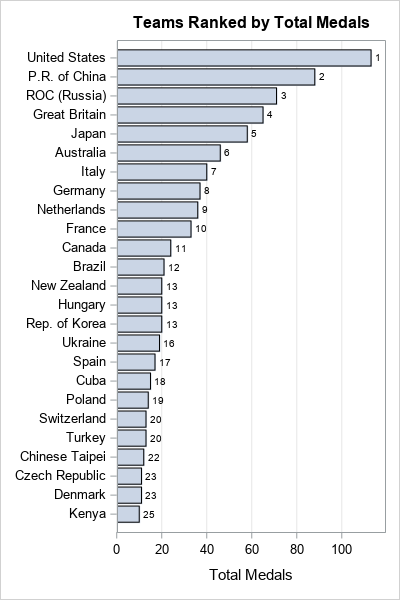The graph displays the total medal count for 25 participating teams. The number to the right of each bar is the rank for the team when you choose equal weights for the medals. This is merely one weighting method that you can use.

Under this set of weights, a team that wins 10 gold medals (and nothing else) gets the same rank as a team that wins 10 bronze medals (and nothing else). To some, this seems unfair to the first team. Consequently, it makes sense to consider weights for which gold is weighted more than silver, which is weighted more than bronze.

### Alternative weighting methods

Let's see how these rankings change if you choose a different set of weights. If you intend to compare different methods, it is useful to scale the weight vectors so that the "total weight" is unity. Thus, you would modify the previous weight vector to w = (1, 1, 1)/3, which is interpreted as "1/3 of the score comes from the number of gold medals, 1/3 comes from the number of silver medals, and 1/3 comes from the number of bronze medals." The following methods are popular choices for weights. For more about methods for weighting medal counts, see Wood, R. (2010) "Comparison of Weighted Ranking Systems."

• Most Medals: w=(1,1,1)/3.
• Most Gold Medals: w=(1,0,0).
• Arithmetic weights: w=(3,2,1)/6. This method values a gold medal as equal to one silver and one bronze.
• Geometric weights: w=(4,2,1)/7. For this method, gold medal equals two silvers. A silver medal equals two bronzes.
• London 1908: w=(5,3,1)/9. This set of weights was used at the 1908 London Games.

You can use the SAS DATA step to compute the scores for each team under each different weighting scheme:

```data WeightedScores; length Wt \$24; set Medals2020; Method=1; Wt="Most Medals: w=(1,1,1)/3"; Score = (1*Gold + 1*silver + 1*Bronze)/3; output; Method=2; Wt="Most Gold: w=(1,0,0)"; Score = 1*Gold + 0*silver + 0*Bronze; output; Method=3; Wt="Arithmetic: w=(3,2,1)/6"; Score = (3*Gold + 2*silver + 1*Bronze)/6; output; Method=4; Wt="Geometric: w=(4,2,1)/7"; Score = (4*Gold + 2*silver + 1*Bronze)/7; output; Method=5; Wt="London 1908: w=(5,3,1)/9"; Score = (5*Gold + 3*silver + 1*Bronze)/9; output; run;   /* Use PROC RANK to run a BY-group analysis for these weighting methods */ proc sort data=WeightedScores; by Method; run; proc rank data=WeightedScores out=Ranks ties=Low descending; by Method; var Score; ranks Rank; run;   /* prepare data for graphing */ proc sort data=Ranks; by Method Order; run;   ods graphics /width=900px height=600px; title "Teams Ranked by Alternative Weighting Schemes"; proc sgpanel data=Ranks; where Method^=1; panelby Wt / novarname rows=1 onepanel sort=data; hbar Team / response=Score datalabel=Rank; colaxis grid label="Weighted Score"; rowaxis discreteorder=data display=(nolabel); run;```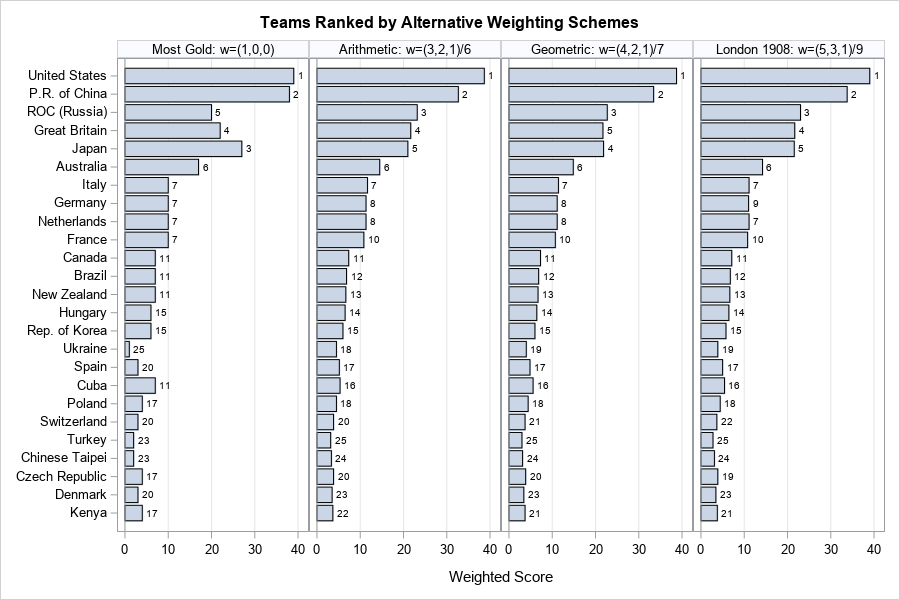Click on the panel to enlarge the graph. In the panel, the teams are listed according to the total number of medals. In each panel, the length of the bar shows the team scores under a different weighting scheme. The numbers to the right of the bars indicate the team ranks. For these data, all methods consistently rank USA and China as the top teams. However, the ranks of other teams move around as you change the weighting criteria. Pay particular attention to teams that have an unequal distribution of medal types, such as Ukraine (1 gold; 6 silver; 12 bronze), Cuba (7 gold, 3 silver, and 5 bronze), and Turkey (2 gold, 2 silver, 9 bronze). For methods that give gold much more weight than bronze, Cuba will rank higher, and Ukraine and Turkey will rank lower, relative to using equal weights.

• If you use the "Most Gold" ranking, Japan's team leapfrogs into third place, followed by the UK. A problem with the "Most Gold" ranking is that it results in many ties. For example, four teams are tied for seventh place. Should Italy (10 gold; 10 silver; 20 bronze) rank the same as France (10 gold; 12 silver; 11 bronze)? Maybe so, maybe not!
• If you use the "Arithmetic Ranking," the teams at the top of the list do not change. It is not until you compute the scores for Cuba and Spain that you start to see teams switch places in the rankings. Teams that move down in the rankings are those who won a relatively large number of bronze medals.
• If you use the "Geometric Ranking," Japan and the UK switch places at the top of the ranks, but mostly the changes occur lower in the rankings. As expected, Ukraine, Cuba, and Turkey are heavily affected.
• The "London 1908" method is very similar to the "Arithmetic" ranking. The London method uses an arithmetic progression of weights, but the step size is 2 instead of 1.

### Summary

Rankings are everywhere. Behind every ranking is a vector of weights that assigns the relative importance of the factors. As demonstrates in these articles, if you change the weights, you will change the scores, which often change the rankings. Consequently, when you see a ranking reported in the media, you should think about what criteria (and biases!) are used to create the ranking. Are they the same criteria that you value? Furthermore, you should realize that a slight tweak to the weights will often change the rankings. A team that is ranked 10th in one set of rankings might be ranked considerably higher or lower if you use slightly different criteria. When the rankings are based on statistical quantities (such as means and proportions), you can add confidence limits that quantify the uncertainty in the rankings.

Although the example uses sports teams, the same analysis is generally applicable for ranking other quantities: stocks, loan applicants, suppliers, job applicants, and so forth.

The post A comparison of different weighting schemes for ranking sports teams appeared first on The DO Loop.People love rankings. You've probably seen articles about the best places to live, the best colleges to attend, the best pizza to order, and so on. Each of these is an example of a ranking that is based on multiple characteristics. For example, a list of the best places to live might base the ranking on quantities such as the average salary, the cost of homes, crime rates, quality of schools, and so forth. Pizzas might be ranked on the crust, the sauce, the toppings, and more. Typically, the ranking is a weighted average of these multiple variables.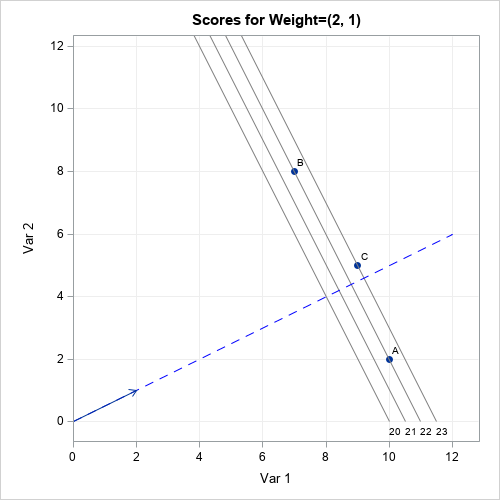Inevitably, after the rankings are released, some people complain that the weights used to construct the ranking were not fair. The most common complaint is that the weights were chosen arbitrarily by the editors. This is a valid criticism: someone must choose the weights, but not everyone will agree with the choice. Some organizations base the weights on surveys in which people are asked to weigh the relative importance of the factors. Other organizations might use equal weights for the characteristics. Others just make up weights that seem to work well.

This article discusses the geometry of a weighted mean (or sum) of multiple variables. When you specify the weights, you are choosing a direction (a line) in the space of the variables. Geometrically, each subject (city, college, pizza, ...) is projected onto the line. The ranking is then determined by the relative placement of each subject when projected onto the line. This is shown visually by the graph to the right. The details of the graph are explained later in this article.

Mathematically, a weighted mean and a weighted sum are very similar and result in the same ranks. I will use the weighted sum in this article. If you prefer, you can get the weighted mean by dividing the weighted sum by the number of factors. Also, a projection onto a line requires scaling the weight vector to have unit length, but I will ignore that technical detail.

### Two-dimensional rankings

To illustrate the geometry of ranking subjects, consider the case where there are only two characteristics. Suppose three students are being evaluated for admission to a university program. Each subject submits two scores on a standardized test. The following table shows the test scores for the three students: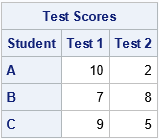Which student is the best fit for the university program? It depends on how the program weights the two test scores. Let's pretend that "Test 1" is a test of mathematical and analytical skills and that "Test 2" is a test of verbal and language skills. Some programs (math, engineering, and other STEM disciplines) will put extra weight on the math score, whereas other programs (creative writing, linguistics, journalism, ...) will put extras weight on the verbal score. Here are a few ways that various programs might weigh the tests:

• A math department might look only at the math test and ignore the language test. This corresponds to the weight vector w1=(1,0).
• A law school might want to equally weigh the math and language tests. This corresponds to the weight vector w2=(1,1).
• An engineering program might decide that math skills are twice as important as language skills. This corresponds to the weight vector w3=(2,1).

The following table displays the weighted sums for each student under these different choices for weights: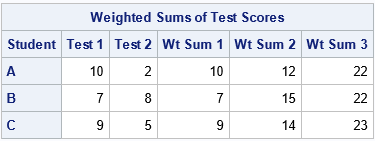The table shows that the "best" candidate depends on the weights that the programs choose:

• For the math department ("Wt Sum 1"), Student A is the best candidate.
• For the law school ("Wt Sum 2"), Student B is the best candidate.
• For the engineering program ("Wt Sum 3"), Student C is the best candidate.

### The geometry of weighted averages

You can plot the test scores as ordered pairs in two-dimensional space. The weight vector determines a line. The projection of the ordered pairs onto that line determines the ranking of the subjects. For example, the following graph shows the geometry for the weight vector w1=(1,0):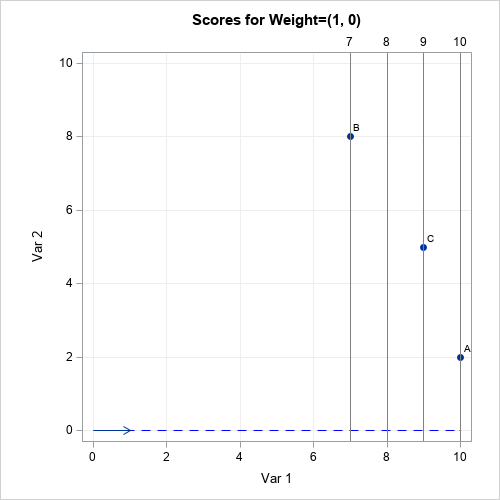For this weight vector, the weighted sum is merely the first component. This is also the projection of the point onto the blue dashed line αw1, for any scalar value of α. The projections that are far from the origin have a larger weighted sum than the projections that are closer to the origin. The students' ranks are determined by their relative positions along the blue dashed line. For this example, Student A is the most highly ranked student (for the math department) because its projection onto the line is farthest from the origin. The gray lines indicate the projection onto the blue line. The gray lines are perpendicular to the blue line.

If you use a different weight vector, you might get a different result. The following graph shows the results for the same students but for the law-school weight vector w2=(1,1). Again, the students' ranks are determined by their relative positions along the blue dashed line. But this time, Student B is the most highly ranked student because its projection onto the line is farthest from the origin. The gray lines are perpendicular to the blue line and indicate the projection onto the blue line.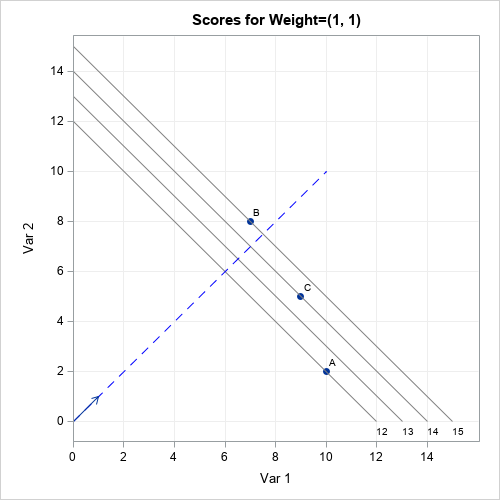The ranks of the students change again if you consider the weights given by w3=(2,1). For these weights, Student C is the highest-ranked student by the engineering school. The graph is shown at the top of this article. Note that students A and B are assigned the same rank because they lie on the same gray line, which is perpendicular to the blue line. This can happen for any weight vector: If two students are on the same perpendicular line, their projection is the same.

### Ranking by using additional variables

The geometry does not change much if you compute a weighted sum of more than two factors. For three factors, the weight vector determines a (blue dashed) line in 3-D space, and the scores are projected onto the line. In the 2-D examples, I drew gray lines perpendicular to the blue line. For three factors, the analogous structures are planes that are perpendicular to the blue line. All subjects on the same plane have the same ranking. In d dimensions, the planes become (d-1)-dimensional hyperplanes.

An important consideration for this process (in any dimension) is that the factors should be measured on comparable scales. In the earlier example, both standardized test scores were assumed to be measured on the same scale. However, you should standardize variables that are on different scales. For example, you would not want to use a weighted average of the median home price (possibly \$300,000) and the mean annual temperature (possibly 15°C) without standardizing those quantities.

### Summary

Sometimes rankings are based on a statistical model, but other times they are based on a "gut feeling" about which features are important. Magazines publish annual rankings of colleges, cities, hospitals, pizzas, and places to work. It is important to realize that the weights that are used for these rankings are somewhat arbitrary. Even if the weights are based on a survey, there is uncertainty in the weights.

This article shows that if you vary the weights, the rankings can change. This article discusses the geometry of weighted sums and averages. A weight vector determines a direction in a d-dimensional feature space. To rank subjects, you project d-dimensional tuples of points onto the line that is determined by the weight vector. The highest-ranked subject is the one that is farthest from the origin along the direction of the weight vector.

So next time you disagree with a ranking, you can confidently say to your friends, "Yes, but if they had used different weights, the results would have been different."

The post Rankings and the geometry of weighted averages appeared first on The DO Loop.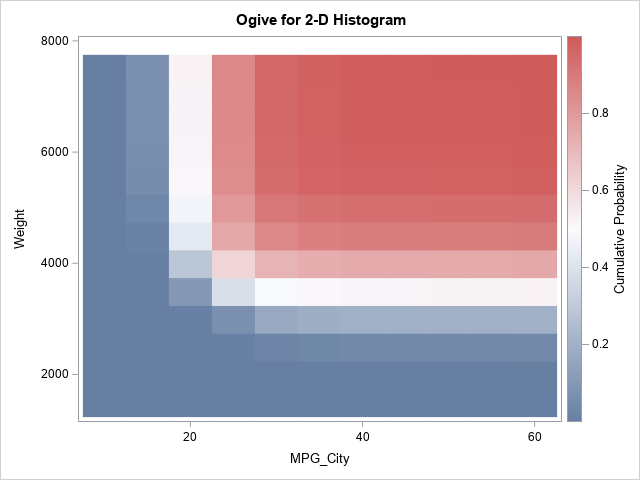A recent article about how to estimate a two-dimensional distribution function in SAS inspired me to think about a related computation: a 2-D cumulative sum. Suppose you have numbers in a matrix, X. A 2-D cumulative sum is a second matrix, C, such that the C[p,q] gives the sum of the cells of X for rows less than p and columns less than q. In symbols, C[p,q] = sum( X[i,j], i ≤ p, j ≤ q ).

This article shows a clever way to compute a 2-D cumulative sum and shows how to use this computation to compute a 2-D ogive, which is a histogram-based estimate of a 2-D cumulative distribution (CDF). An example of a 2-D ogive is shown to the right. You can compare the ogive to the 2-D CDF estimate from the previous article.

### 2-D cumulative sums

A naive computation of the 2-D cumulative sums would use nested loops to sum submatrices of X. If X is an N x M matrix, you might think that the cumulative sum requires computing N*M calls to the SUM function. However, there is a simpler computation that requires only N+M calls to the CUSUM function. You can prove by induction that the following algorithm gives the cumulative sums:

1. Compute the cumulative sums for each row. In a matrix language such as SAS/IML, this requires N calls to the CUSUM function.
2. Use the results of the first step and compute the "cumulative sums of the cumulative sums" down the columns. This requires M calls to the CUSUM function.

Thus, in the SAS/IML language, a 2-D cumulative sum algorithm looks like this:

```proc iml; /* compute the 2-D CUSUM */ start Cusum2D(X); C = j(nrow(X), ncol(X), .); /* allocate result matrix */ do i = 1 to nrow(X); C[i,] = cusum( X[i,] ); /* cusum across each row */ end; do j = 1 to ncol(X); C[,j] = cusum( C[,j] ); /* cusum down columns */ end; return C; finish; store module=(Cusum2D);   Count = { 7 6 1 3, 6 3 5 2, 1 2 4 1}; Cu = Cusum2D(Count); print Count, Cu;```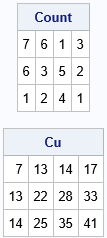The output shows the original matrix and the 2-D cumulative sums. For example, in the CU matrix, the (2,3) cell contains the sum of the submatrix Count[1:2, 1:3]. That is, the value 28 in the CU matrix is the sum (7+6+1+6+3+5) of values in the Count matrix.

### From cumulative sums to ogives

Recall that an ogive is a cumulative histogram. If you start with a histogram that shows the number of observations (counts) in each bin, then an ogive displays the cumulative counts. If you scale the histogram so that it estimates the probability density, then the ogive estimates the univariate CDF.

A previous article showed how to construct an ogive in one dimension from the histogram. To construct a two-dimensional ogive, do the following:

• You start with a 2-D histogram. For definiteness, let's assume that the histogram displays the counts in each two-dimensional bin.
• You compute the 2-D cumulative sums, as shown in the previous section. If you divide the cumulative sums by the total number of observations, you obtain the cumulative proportions.
• The matrix of cumulative proportions is the 2-D ogive. However, by convention, an ogive starts at 0. Consequently, you should append a row and column of zeros to the left and top of the matrix of bin counts.

You can download the complete SAS/IML program that computes the ogive. The program defines two SAS/IML functions. The Hist2D function creates a matrix of counts for bivariate data. The Ogive2D function takes the matrix of counts and converts it to a matrix of cumulative proportions by calling the Cusum2D function in the previous section. It also pads the cumulative proportions with a row and a column of zeros. The function returns a SAS/IML list that contains the ogive and the vectors of endpoints for the ogive. The following statements construct an ogive from the bivariate data for the MPG_City and Weight variables in the Sashelp.Cars data set:

```proc iml; /* load computational modules */ load module=(Hist2D Cusum2D Ogive2D);   /* read bivariate data */ use Sashelp.Cars; read all var {MPG_City Weight} into Z; close;   * define cut points in X direction (or use the GSCALE function); cutX = do(10, 60, 5); cutY = do(1500, 7500, 500); Count = Hist2D(Z, cutX, cutY); /* matrix of counts in each 2-D bin */ L = Ogive2D(Count, cutX, cutY); /* return value is a list */ ogive = L\$'Ogive'; /* matrix of cumulative proportions */ print ogive[F=Best5. r=(L\$'YPts') c=(L\$'XPts')];```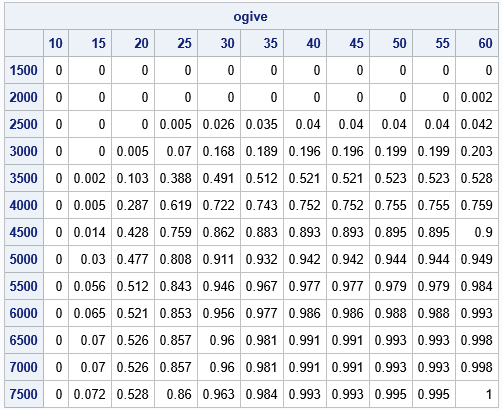The table shows the values of the ogive. You could use bilinear interpolation if you want to estimate the curve that corresponds to a specific probability, such as 0.5. You can also visualize the ogive by using a heat map, as shown at the top of this article. Notice the difference between the tabular and graphical views: rows increase DOWN in a matrix, but the Y-axis points UP in a graph. To compare the table and graph, you can reverse the rows of the table.

### Summary

This article shows how to compute a 2-D cumulative sum. You can use the 2-D cumulative sum to create a 2-D ogive from the counts of a 2-D histogram. The ogive is a histogram-based estimate of the 2-D CDF. You can download the complete SAS program that computes 2-D cumulative sums, histograms, and ogives.

The post Compute 2-D cumulative sums and ogives appeared first on The DO Loop.Many nonparametric statistical methods use the ranks of observations to compute distribution-free statistics. In SAS, two procedures that use ranks are PROC NPAR1WAY and PROC CORR. Whereas the SPEARMAN option in PROC CORR (which computes rank correlation) uses only the "raw" tied ranks, PROC NPAR1WAY uses transformations of the ranks, called scores, to compute rank-based analysis of variance (ANOVA), empirical distribution tests, and other analyses. This article shows two things:

• How to generate normally distributed scores that have the same (rank) correlation as the original data.
• How these scores are computed if there are tied values in the data.

### What is a "score" in statistics?

Statisticians like the word "score." As a verb, "to score" means to evaluate a regression model on one or more observations. As a noun, "raw score" means the original data. "Standardized score" (or z-score) implies that the data have been transformed according to a standardizing transformation. The "rank score" (or Wilcoxon score) is the result of replacing each observation by its rank. Other types of rank-based scores, often called normal scores, are explained in the documentation for PROC NPAR1WAY, including the Blom, Tukey, and van der Waerden scores.

### Ranks and normal scores

You can use the NORMAL= option in PROC RANK to obtain three common rank-based normal scores. The three scores are essentially the same and are can be used to construct normal Q-Q plots. Assume that the data do not have any duplicate values. Let Ri be the rank of the i_th observation of a data vector, X. Then the normal scores have the form
si = Φ-1( (Ri−c) / (n+b) )
where Φ-1 is the inverse standard cumulative normal distribution, and n is the number of nonmissing observations.

• For Blom's score, c=3/8 and b=1/4
• For Tukey's score, c=1/3 and b=1/3
• For van der Waerden's score, c=0 and b=1

If the data have duplicate values, you need to adjust the formulas slightly, as shown in the last section of this article.

### Why are normal scores useful?

The idea behind a normal score is rather clever. You compute the ranks of each variable and scale them so that they are in the range (0,1). You then compute the standard normal quantiles of these values. The resulting scores are often approximately normal.

Because the transformation includes a standardization, some statistics (such as the median and the interquartile range) are different between the data and the scores. However, the ranks of the scores are the same as the ranks of the original data, which means that many rank-based statistics are identical for the data and for the scores. This includes multivariate statistics such as the rank correlation between two variables.

Let's compute an example. First, let's compute the rank correlation between three variables in the Sashelp.Cars data set. The variables are not normally distributed, as shown by the matrix of scatter plots and the marginal histograms:

```proc corr data=Sashelp.Cars spearman nosimple noprob plots(maxpoints=none)=matrix(hist); var EngineSize MPG_Highway Weight; label EngineSize= MPG_Highway= Weight=; /* suppress labels */ run;```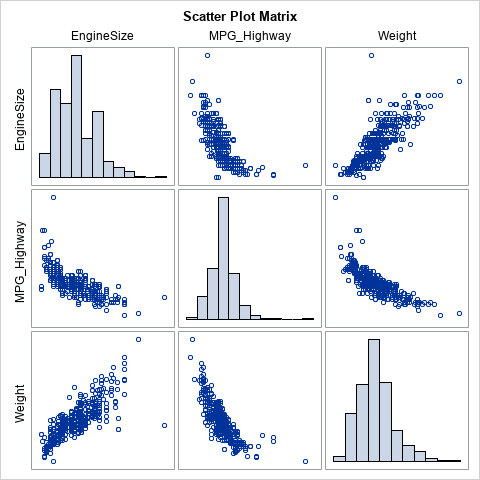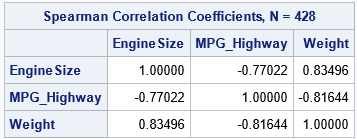You can use PROC RANK to create new variables that contain the normal scores for the variables. The NORMAL= option supports all three normal scores. For this example, I will use the van der Waerden scores (NORMAL=VW). The following statements generate the variables, then compute the rank correlation between the scores:

```/* write VDW scores to RANKOUT data set */ proc rank data=Sashelp.Cars out=RankOut ties=mean normal=VW; var EngineSize MPG_Highway Weight; ranks vdwEngineSize vdwMPG_Highway vdwWeight; /* names of scores */ run;   proc corr data=RankOut spearman nosimple noprob plots(maxpoints=none)=matrix(hist); var vdw:; label vdwEngineSize= vdwMPG_Highway= vdwWeight=; /* suppress labels */ run;```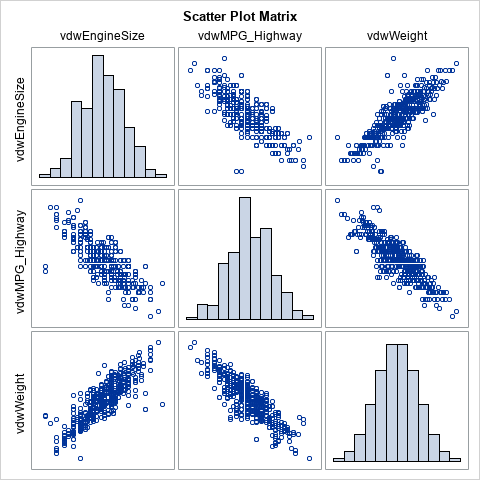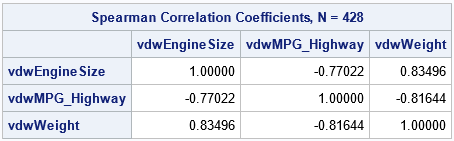The rank correlations between the scores are exactly the same as for the original data. This is because the transformation between data and scores preserves the ranks. The scatter plot matrix of the scores shows that the scores are approximately multivariate normal. The bivariate scatter plots show the elliptical shapes that you would expect for correlated multivariate data.

This example shows the value of transforming data to scores. You get a new set of variables that are approximately multivariate normal and that have the same (rank) correlation as the original data. Although the Pearson product-moment correlation is not invariant under the transformation, the Pearson and Spearman correlations tend to be close to each other, so the Pearson correlation for the data tends to be close to the Spearman correlation for the scores.

### Compute a normal score for tied values

As mentioned previously, when there are tied values, the transformation to produce the normal scores needs to be modified. You might guess that you should compute the tied ranks and then transformed the tied ranks into normal scores. However, both PROC NPAR1WAY and PROC RANK do something slightly different. The following quote is from the documentation for PROC NPAR1WAY:

When there are tied values, PROC NPAR1WAY first sorts the observations in ascending order and assigns ranks as if there were no ties. Then the procedure computes the scores based on these ranks by using the formula for the specified score type. The procedure averages the scores for tied observations and assigns this average score to each of the tied observations. Thus, all equal data values have the same score value.

The following SAS/IML program computes the van der Waerden scores for the three variables in the Sashelp.Cars data. First, the program computes the scores "incorrectly" by using the RANKTIE function to compute the averaged ranks and then transforming the tied ranks. Next, the program uses the method in the PROC NPAR1WAY documentation to compute the correct scores. Both methods are compared to the output from PROC RANK. The numerical difference between the incorrect-but-fast method and the correct-but-slower method is very small.

```proc iml; /* read the original data */ varNames = {'EngineSize' 'MPG_Highway' 'Weight'}; use Sashelp.Cars; read all var varNames into Y; run;   /* The simple method: If there are no ties, these statements transform the data into the van der Waerden scores. Assume Y does not have any missing values. */ n = nrow(Y); SimpleScores = j(n, ncol(Y), .); do i = 1 to ncol(Y); r = ranktie(Y[,i], "mean"); SimpleScores[,i] = quantile("Normal", r/(n+1)); end;   /* read the scores from PROC RANK and compare */ use RankOut; read all var {'vdwEngineSize' 'vdwMPG_Highway' 'vdwWeight'} into VDW; close;   maxDiffSimple = max(abs(SimpleScores-VDW));   /* If tied values, the computation is different and more complicated. */ start VDWTies(X); n = nrow(X); rank = rank(X); /* ignore ties */ q = quantile("Normal", rank/(n+1)); /* compute VDW scores */ score = j(n,1,.); uX = unique(X); /* get the distinct values */ do i = 1 to ncol(uX); /* for each distinct value */ idx = loc( X=uX[i] ); /* locate all ties for this value */ score[idx] = mean(q[idx]); /* average the scores */ end; return score; finish;   scores = j(n, ncol(Y), .); do j = 1 to ncol(Y); scores[,j] = VDWTies(Y[,j]); end;   maxDiff = max(abs(scores-VDW)); print maxDiffSimple maxDiff;```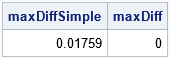The simple application of the van der Waerden formula is slightly different from the PROC RANK output. To get the correct scores, you must average the scores of the tied values. In practice, you can often use the simpler transformation, especially for large samples and for variables that have only a small proportion of tied values.

Even for moderate-sized samples (this example has 428 observations), the two methods tend to agree. For these data, the EngineSize variable has only 43 unique values. The MPG_Highway variable has only 33 unique values. Nevertheless, the simple computation of the van der Waerden scores is almost the same as the more complicated method that is used by PROC NPAR1WAY. A scatter plot of the two scores shows that the scores computed by the two methods are almost the same. The following statements create the scatter plot for the MPG_Highway variable:

```title "MPG_Highway Variable"; call scatter(SimpleScores[,2], scores[,2]) procopt="noautolegend aspect=1" label={'Simple Scores' 'Tied Scores'} lineparm={0 0 1};```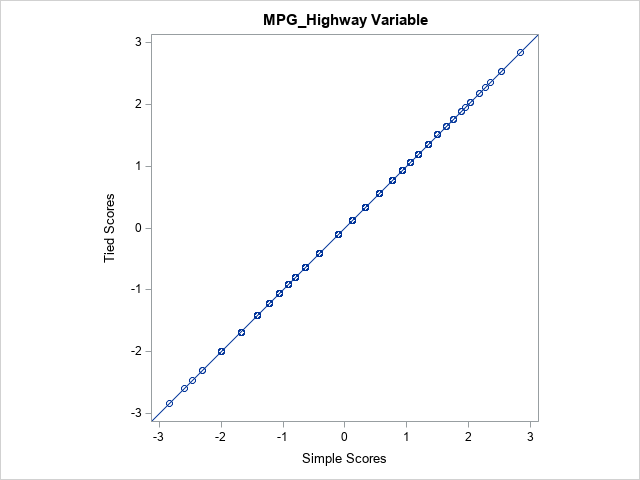### Summary

This article discusses rank-based normal scores, which are used in certain nonparametric tests. The scores are obtained by transforming the ranks of the original data. The scores have the same ranks as the data. Furthermore, the scores are approximately normally distributed, and they have the same rank correlation as the data.

This article also shows the correct way to compute the normal scores for tied values in the data. However, there is often little difference between the true scores and a simpler approximation that transforms the tied ranks.

The post Rank-based scores and tied values appeared first on The DO Loop.For many univariate statistics (mean, median, standard deviation, etc.), the order of the data is unimportant. If you sort univariate data, the mean and standard deviation do not change. However, you cannot sort an individual variable (independently) if you want to preserve its relationship with other variables. This statement is both obvious and profound. For example, the correlation between two variables X and Y depends on the (X,Y) pairs. If you sort Y independently and call the vector of sorted observations Z, then corr(X,Z) is typically not equal to corr(X,Y), even though Y and Z contain exactly the same data values.

This observation is the basis behind permutation tests for paired data values. In a permutation test, you repeatedly change the order of one of the variables independently of the other variable(s). The goal of a permutation test is to determine whether the original statistic is likely to be observed in a random pairing of the data values.

This article looks at how sorting one variable (independently of another) changes the correlation between two variables. You can use this technique to construct a new vector that has the same values (marginal distribution) but almost any correlation you want with the other vector.

### A simple permutation: A sorted vector of values

The Sashelp.Class data set in SAS contains observations about the height, weight, age, and gender of 19 students. The Height and Weight variables are highly correlated (R = 0.88), as shown by the following call to PROC CORR in Base SAS:

```data class; set Sashelp.Class; keep Height Weight; run;   proc corr data=Class nosimple; var Height Weight; run;```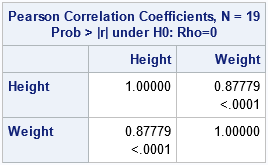The output from PROC CORR computes the sample correlation between the Height and Weight variables as 0.88. The p-value is highly significant (p < .0001), which indicates that it would be rare to observe a statistic this large if the heights and weights were sampled randomly from an uncorrelated bivariate distribution.

Consider the following experiment: What happens if you sort the second variable and look at the correlation between the first variable and the sorted copy of the second variable? The following program runs the experiment twice, first with Weight sorted in increasing order, then again with Weight sorted in decreasing order:

```/* sort only the second variable: First increasing order, then decreasing order */ proc sort data=Class(keep=Weight) out=Weight1(rename=(Weight=WeightIncr)); by Weight; run; proc sort data=Class(keep=Weight) out=Weight2(rename=(Weight=WeightDecr)); by descending Weight; run;   /* add the sorted variables to the original data */ data Class2; merge Class Weight1 Weight2; run; /* what is the correlation between the first variable and permutations of the second variable? */ proc corr data=Class2 nosimple; var Weight WeightIncr WeightDecr; with Height; run;```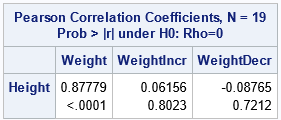The output shows that the original variables were highly correlated. However, if you sort the weight variable in increasing order (WeightIncr), the sorted values have 0.06 correlation with the height values. This correlation is not significantly different from 0. Similarly, if you sort the Weight variable in decreasing order (WeightDecr), the sorted values have -0.09 correlation with the Height variable. This correlation is also not significantly different from 0.

It is not a surprise that permuting the values of the second variable changes the correlation with the first variable. After all, correlation measures how the variables co-vary. For the original data, large Height values tend to be paired with high Weight values. Same with the small values. Changing the order of the Weight variable destroys that relationship.

### A permutation test for correlation

The experiment in the previous section demonstrates how a permutation test works for two variables. You start with pairs of observations and a statistic computed for those pairs (such as correlation). You want to know whether the statistic that you observe is significantly different than you would observe in random pairings of those same variables. To run a permutation test, you permute the values of the second variable, which results in a random pairing. You look at the distribution of values of the statistic when the values are paired randomly. You ask whether the original statistic is likely to have resulted from a random pairing of the values. If not, you reject the null hypothesis that the values are paired randomly.

You can use SAS/IML software and the RANPERM function to create a permutation test for correlation, as follows:

```proc iml; use Class2; read all var "Height" into X; read all var "Weight" into Y; close;   start CorrCoeff(X, Y, method="Pearson"); return ( corr(X||Y, method)[1,2] ); /* CORR returns a matrix; extract the correlation of the pair */ finish;   /* permutation test for correlation between two variables */ call randseed(12345); /* set a random number seed */ B = 10000; /* total number of permutations */ PCorr = j(B, 1, .); /* allocate vector for Pearson correlations */ PCorrObs = CorrCoeff(X, Y); /* correlation for original data */ PCorr = PCorrObs; do i = 2 to B; permY = ranperm(Y)`; /* permute order of Y */ PCorr[i] = CorrCoeff(X, permY); /* correlation between X and permuted Y */ end;```

The previous program computes a vector that has B=10,000 correlation coefficients. The first element in the vector is the observed correlation between X and Y. The remaining elements are the correlations between X and randomly permuted copies of Y. The following statement estimates the p-value for the null hypothesis that X and Y have zero correlation. The two-sided p-value is computed by counting the proportion of the permuted data vectors whose correlation with X is at least as great (in magnitude) as the observed statistic. You can also use a histogram to visualize the distribution of the correlation coefficient under the null hypothesis:

```pVal = sum( abs(PCorr)>= abs(PCorrObs) ) / B; /* proportion of permutations with extreme corr */ print pVal;   title "Permutation Test with H0:corr(X,Y)=0"; title2 "Distribution of Pearson Correlation (B=1000)"; refStmt = "refline " + char(PCorrObs) + " / axis=x lineattrs=(color=red);"; call histogram(PCorr) other=refStmt label="Pearson Correlation";```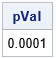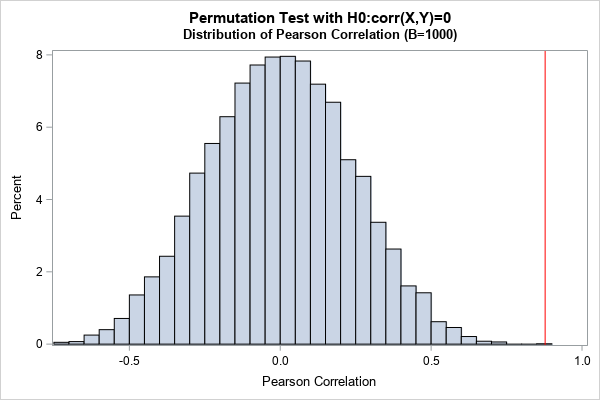The graph and p-value give the same conclusion: It is unlikely that the observed statistic (0.88) would arise from a random pairing of the X and Y data values. The conclusion is that the observed correlation between X and Y is significantly different from zero. This conclusion agrees with the original PROC CORR analysis.

### Find a vector that has a specified correlation with X

Every correlation coefficient in the previous histogram is the result of a permutation of Y. Each permutation has the same data values (marginal distribution) as Y, but a different correlation with X. An interesting side-effect of the permutation method is that you can specify a value of a correlation coefficient that is in the histogram (such as -0.3) and find a vector Z such that Z has the same marginal distribution as Y but corr(X,Z) is the specified value (approximately). The following SAS/IML statements use random permutations to find a data vector with those properties:

```/* Note: We can find a vector Z that has the same values as Y but has (almost) any correlation we want! Choose the target correlation from the histogram, such as r=-0.3. Then find a vector Z that has the same values as Y but has corr(X,Z) = -0.3 */ targetR = -0.3; isMatch = 0; do i = 1 to B while(isMatch=0); /* to avoid an infinite loop, limit search to B permutations */ Z = ranperm(Y)`; /* permute order of Y */ r = CorrCoeff(X, Z); /* correlation between X and permuted Y */ isMatch = ( abs(r - targetR) < 0.01 ); /* is correlation close to target? */ end;   print r; title "X versus Permutation of Y"; call scatter(X,Z);```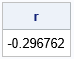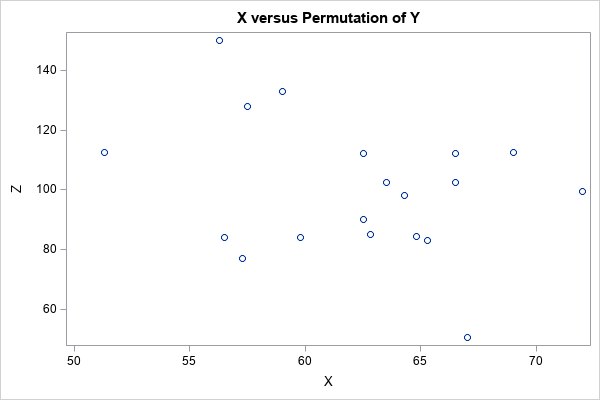The scatter plot shows the bivariate distribution of X and Z, where Z has the same data values as Y, but the correlation with X is close to -0.3. You can choose a different target correlation (such as +0.25) and repeat the experiment. If you do not specify a target correlation that is too extreme (such as greater than 0.6), this method should find a data vector whose correlation with X is close to the specified value.

Notice that not all correlations are possible. There are at most n! permutations of Y, where n is the number of observations. Thus, a permutation test involves at most n! distinct correlation coefficients.

This article shows that permuting the data for one variable (Y) but not another (X) destroys the multivariate relationship between X and Y. This is the basis for permutations tests. In a permutation test, the null hypothesis is that the variables are not related. You test that hypothesis by using random permutations to generate fake data for Y that are unrelated to X. By comparing the observed statistic to the distribution of the statistic for the fake data, you can reject the null hypothesis.

An interesting side-effect of this process is that you can create a new vector of data values that has the same marginal distribution as the original data but has a different relationship with X. In a future article, I will show how to exploit this fact to generate simulated data that have a specified correlation.

The post Permutation tests and independent sorting of data appeared first on The DO Loop.It is well known that classical estimates of location and scale (for example, the mean and standard deviation) are influenced by outliers. In the 1960s, '70s, and '80s, researchers such as Tukey, Huber, Hampel, and Rousseeuw advocated analyzing data by using robust statistical estimates such as the median and the median absolute deviation (MAD) from the median. A common theme in this research was to use robust estimates to identify and exclude outliers. If you are not familiar with the MAD statistic, see the Appendix at the end of this article for an overview and references.

This article discusses an outlier-detection method in time series analysis called the Hampel identifier. It uses robust moving estimates to identify outliers in a time series. If the method identifies an outlier, you might decide to replace the extreme value with an imputed value, such as the rolling median at that time point. This kind of imputation is known as the Hampel filter.

### Detecting outliers: The classical approach

Suppose you have a time series that might have outliers in it. A simple method to detect outliers is to estimate the rolling center of the time series by fitting a smooth curve to the series. You can then classify an observation as an outlier if it is sufficiently far away from the curve. For example, you might classify an outlier as a point, y[t], such that |y[t]- mean[t]| > 3*std[t], where mean[t]is the moving average at t (using some window length) and std[t]is the moving standard deviation.

Unfortunately, the classical moving average and moving standard deviation are influenced by the outliers. Consequently, you might not be able to detect the outliers if you use a moving average as the smoother and use a moving standard deviation to determine "far away." The following example shows how classical estimates can fail to detect outliers. In the example, most observations are on a sine curve except for four outliers that are artificially set to 5, which is far from the sine curve. The examples use PROC EXPAND in SAS/ETS and windows of length 7 to compute a running mean and a running standard deviation:

```data Test; pi = constant('pi'); do t = 1 to 30; y = sin(2*pi*t/30); if t in (3 12 13 24) then y = 5; output; end; run;   proc expand data=Test out=Classical method=NONE; id t; convert y = RunMean / transformout=(cmovave 7); convert y = RunStd / transformout=(cmovstd 7); run;   data Classical; set Classical; lower = RunMean - 3*RunStd; /* classical intervals: mean +/- 3*std */ upper = RunMean + 3*RunStd; run;   title "Moving Average and Standard Deviation"; proc sgplot data=Classical; band x=t lower=lower upper=upper / name="b" legendlabel="Classical Interval"; scatter x=t y=y / name="data"; series x=t y=RunMean / name="c" legendlabel="Running Mean(7)"; keylegend "b" "c"; run;```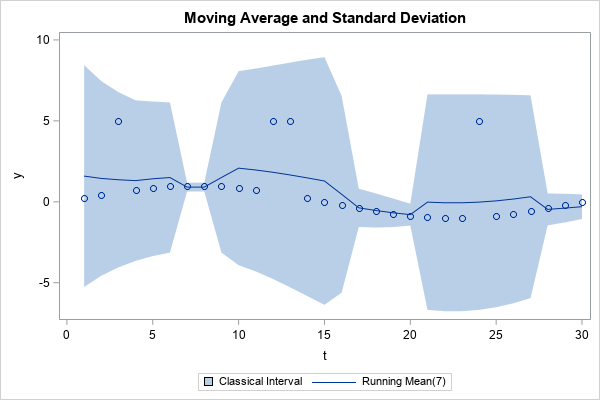The graph overlays the data and a simple centered moving average. The shaded region shows the intervals mean[t]± 3*std[t], where mean[t]and std[t]are the moving average and moving standard deviation, respectively, at time t. You can see that the moving average is higher near the outliers. In addition, the moving standard deviation is larger near the outliers. Because of these two facts, the outliers at t={3 12 13 24} are actually INSIDE the intervals mean[t]± 3*std[t]. Thus, when you use classical (non-robust) estimates, this technique does not detect the outliers.

### The Hampel identifier

If you replace the moving average and moving standard deviation with their robust counterparts (median and MAD), you obtain the Hampel identifier. Hampel suggested that observations that lie outside the moving interval median[t]± h*(1.4826*MAD[t]) be considered outliers. Most practitioners use h=3, but you can use higher values to identify more extreme outliers. The factor 1.4826 is explained in the Appendix to this article. It is chosen so that 1.4826*MAD estimates the standard deviation of normally distributed data.

A previous article shows how to use SAS/IML to compute the points in moving windows of a time series. That article defines the extractWindows function, which returns a matrix whose columns are the data in each moving window. The MAD function in SAS/IML computes the MAD of each column, so you can easily form the Hampel intervals and test which observations are outside of the intervals.

The following SAS/IML program reads the Test data and applies the Hampel identifier. The vector Outlier is a binary indicator variable; the value is 1 for observations that are outside of the robust Hampel intervals:

```proc iml; /* define or STORE/LOAD the extractWindows function. The function is defined at https://blogs.sas.com/content/iml/2021/05/26/running-median-smoother.html */ load module = extractWindows; use Test; read all var {'t' 'y'}; close;   M = extractWindows(y, 3); /* k=3 ==> window length=7 */ med = T( median(M) ); /* column vector of medians */ Nmad = T( mad(M, "NMAD") ); /* column vector of 1.4826*MADs */ /* Hampel identifier: Which obs are outside med +/- 3*NMAD? */ lower = med - 3*Nmad; upper = med + 3*Nmad; outlier = ( abs(y-med) > 3*Nmad );   create Robust var {'t' 'y' 'med' 'lower' 'upper' 'outlier'}; append; close; QUIT;   title "Robust Moving Estimates"; title2 "Hampel Filter Using Median and MAD"; proc sgplot data=Robust; band x=t lower=lower upper=upper / name="b" legendlabel="Robust Interval"; scatter x=t y=y / name="group" group=outlier; series x=t y=med / name="c" legendlabel="Running Median(7)"; keylegend "b" "c"; run;```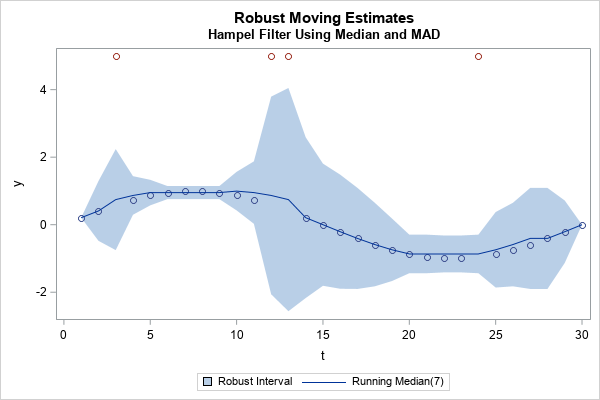Unlike the classical method, the robust Hampel identifier successfully flags all four unusual observations. Notice two features of the graph:

• The curve of rolling medians is closer to the underlying sine curve than the curve for rolling means.
• The robust Hampel intervals (the shaded region) are narrower than the corresponding classical intervals. The Hampel intervals are wider near the outliers (as they should be) but are small enough that the unusual observations are outside the intervals.

### The Hampel identifier for real data

How well does the Hampel identifier work on real data? The previous article included data for the temperature of a cow. The following program reads the COWS data and uses the Hampel identifier to locate unusual temperatures:

```/* apply the Hampel identifier to the Cows data */ proc iml; /* define or STORE/LOAD the extractWindows function. */ load module = extractWindows; use Cows; read all var {Day y}; close;   /* Hampel identifier: Use moving median and MAD to identify outliers */ start HampelID(y, k, multiplier=3); M = extractWindows(y, k, "REPEAT"); med = T( median(M) ); Nmad = T( mad(M, "NMAD") ); idx = loc( abs(y-med) > multiplier*Nmad ); return idx; finish;   idx = HampelID(y, 3); print idx;   outlier = j(nrow(y), 1, 0); /* most obs are not outliers */ outlier[idx] = 1; /* these were flagged as potential outliers */```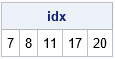For the COWS data, the observations on days {7, 8, 11, 17, 20} are identified by the Hampel intervals as potential outliers. You can overlay the running median and Hampel intervals on the COWS data to obtain the following graph: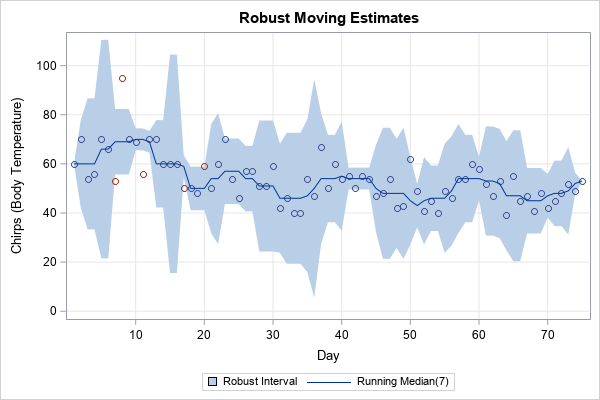The graph verifies that the temperatures on days {7, 8, 11} are unusual. The temperatures on days {17, 20} are marginally outside of the Hampel intervals. As with all algorithms that identify potential outliers, you should always examine the potential outliers, especially if you plan to discard the observations or replace them with an imputed value.

### The Hampel filter

Some practitioners in signal processing use the Hampel identifier to implement the Hampel filter. The filter replaces observations that are flagged as outliers with the rolling median. For example, the following SAS/IML code snippet shows how to replace outliers with the median values:

```/* Hampel filter: Replace outliers with the medians */ newY = y; /* copy old series */ idx = loc(outlier); /* find the indices where outlier=1 */ if ncol(idx)>0 then /* are there any outliers? */ newY[idx] = med[idx]; /* replace each outlier with the rolling median */```

### Summary

This article shows how to implement the Hampel identifier in SAS. The Hampel identifier uses robust moving estimates (usually the rolling median and rolling MAD) to identify outliers in a time series. If you detect an outlier, you can replace the extreme value by using the rolling median, which is a process known as the Hampel filter.

This section briefly discusses the median absolute deviation (MAD) statistic, which you can think of as a robust version of the standard deviation. For univariate data X={x1, x2, ..., xL}, the MAD is computed by computing the median (m = median(X)) and the median of the absolute deviations from the median: MAD = median(|x_i - m|). You can compute the MAD statistic (and other robust estimates of scale) by using the ROBUSTSCALE option in PROC UNIVARIATE, as follows:

```proc univariate data=Test robustscale; var y; ods select robustscale; run;```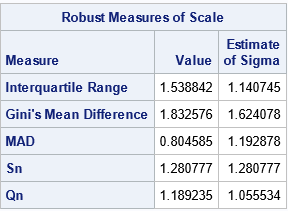SAS also supports the MAD function in the DATA step and in PROC IML. If you are using a moving statistic, the MAD is applied to the data in each rolling window.

For a normal population, the MAD is related to σ, the population standard deviation, by the formula σ ≈ 1.4826*MAD. Therefore, if you have normally distributed data, you can use 1.4826*MAD to estimate the standard deviation. (The scale factor 1.4826 is derived in the Wikipedia article about MAD.) For example, the following SAS/IML program generates 10,000 random observations from a standard normal distribution. It computes the sample standard deviation and MAD statistics. The "NMAD" option automatically multiplies the raw MAD by the appropriate scale factor. You can see that the scaled MAD is close to the standard deviation for these simulated data:

```proc iml; call randseed(4321); x = randfun(10000, "Normal"); sd = std(x); /* should be close to 1 */ /* for normally distributed data, 1.4826*MAD is a consistent estimator for the standard deviation. */ madN = mad(x, "NMAD"); /* = 1.4826*mad, which should be close to 1 */ print sd madN;```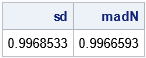The post The Hampel identifier: Robust outlier detection in a time series appeared first on The DO Loop.When data contain outliers, medians estimate the center of the data better than means do. In general, robust estimates of location and sale are preferred over classical moment-based estimates when the data contain outliers or are from a heavy-tailed distribution. Thus, instead of using the mean and standard deviation of data, some analysts prefer to use robust statistics such as the median, the trimmed mean, the interquartile range, and the median absolute deviation (MAD) statistic.

A SAS statistical programmer recently wanted to use "rolling" robust statistics to analyze a time series. The most familiar rolling statistic is the moving average, which is available in PROC EXPAND in SAS/ETS software. In addition to rolling weighted means, the EXPAND procedure supports a rolling median, which is shown in the next section. However, it does not compute a robust rolling estimate of scale, such as a rolling MAD statistic. However, you can use PROC IML to compute robust estimates.

This article discusses how to compute robust rolling statistics for a time series. A subsequent article will discuss the rolling MAD statistic, which enables you to identify outliers in a time series.

### An example of a rolling median

The following example shows how to compute a running median in SAS. The data (from Velleman and Hoaglin, 1981, p. 161ff) are the daily temperature of a cow (measured by counting chirps from a telemetric thermometer). Peak temperatures are associated with fertility, so dairy farmers want to estimate the peaks of the time series to predict the best time to impregnate the cows. One simple technique is to compute the rolling median. Although a farmer would probably use a rolling window that looks back in time (such as the median of the last seven days), this example uses a seven-day centered time window to compute the rolling median:

```/* Daily temperature of a cow at 6:30 a.m. measured by counting chirps from a telemetric thermometer implanted in the cow. y = (chirps per 5-minute interval) - 800. Peak temperatures are associated with fertility. Cows peak about every 15-20 days. Source: Velleman and Hoaglin (1981). */ data Cows; input y @@; Day + 1; datalines; 60 70 54 56 70 66 53 95 70 69 56 70 70 60 60 60 50 50 48 59 50 60 70 54 46 57 57 51 51 59 42 46 40 40 54 47 67 50 60 54 55 50 55 54 47 48 54 42 43 62 49 41 45 40 49 46 54 54 60 58 52 47 53 39 55 45 47 41 48 42 45 48 52 49 53 ;   proc expand data=Cows out=MedOut method=NONE; id Day; convert y = RunMed / transformout=(cmovmed 7); /* Centered MOVing MEDian on a 7-day window */ run;   title "Overlay Running Median"; title2 "Window Length = 7"; proc sgplot data=MedOut noautolegend; scatter x=Day y=y; series x=Day y=RunMed; xaxis grid values=(0 to 80 by 10); yaxis grid label="Chirps (Body Temperature)"; run;```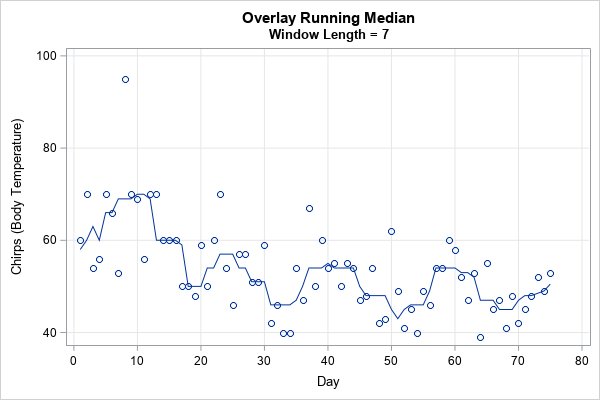The graph overlays the rolling median on the time series of the cow's temperature. Days 10, 24, 40, and 60 are approximate peaks of the cow's temperature and are therefore good days for the farmer to impregnate this cow. The moving median smooths the cow's daily temperatures and makes the trends easier to visualize. Furthermore, a MOOO-ving median is easy to cow-culate.

### Compute rolling windows in SAS/IML

I previously showed how to implement rolling statistics in the SAS/IML language. The typical technique is to

• For each time point, extract the data within the time window for that time point. For example, the previous example uses a seven-day centered window.
• Compute statistics for the data in each window.

If you want to compute several statistics on the same data, it can be advantageous to form a matrix that contains the data in each window. If the time series contains n observations, and each window contains L data values, then you can form the L x n matrix whose columns contain the data in the windows. You can then compute multiple statistics (means, standard deviations, medians, MADs, ....) on the columns of the matrix. For centered windows, you must decide what data to use for the first few and last few data points in the series. Two popular choices are to use missing values or to repeat the first and last points. I use the term Tukey padding to indicate that a series has been padded by repeating the first and last values.

### Extract data for each window

This section shows one way to extract the data in the n windows. The following SAS/IML function pads the time series by using a user-defined value (usually a missing value) or by using Tukey padding. It then forms the (2*k+1) x n matrix whose i_th column is the window of radius k about the point y[i]:

```proc iml; /* extract the symmetric sequence about x[i], which is x[i-k-1:i+k+1] if 1 <= i-k-1 and i+k+1 <= nrow(x). Otherwise, pad the sequence. You can pad with a user-defined value (such as missing or zero) or you can repeat the first or last value. This function loops over the number of columns and extracts each window. INPUT: y is the time series k is the radius of the window. The window length is 2*k+1 centered at y[i] padVal can be missing or a character value. If character, use y to pad on the left and y[n] to pad on the right. */ start extractWindows(y, k, padVal="REPEAT"); n = nrow(y); M = j(2*k+1, n, .); /* create new series, z, by padding k values to the ends of y */ if type(padVal)='N' then z = repeat(padVal, k) // y // repeat(padVal, k); /* pad with user value */ else z = repeat(y, k) // y // repeat(y[n], k); /* Tukey's padding */ do i = 1 to n; range = i : i+2*k; /* centered at k+i */ M[,i] = z[range]; end; return M; finish;   /* create a sample time series and extract windows of length=7 (so k=3) */ t = 1:10; y = T(20:29); /* the time series is 20, 21, ..., 28, 29 */ M = extractWindows(y, 3, .); /* missing value padding */ rowlabls = {'x_{t-3}' 'x_{t-2}' 'x_{t-1}' 'x_t' 'x_{t+1}' 'x_{t+2}' 'x_{t+3}'}; /* numeric colnames are supported in SAS/IML 15.1 */ print M[c=t r=rowlabls L="Window of Length=7"];```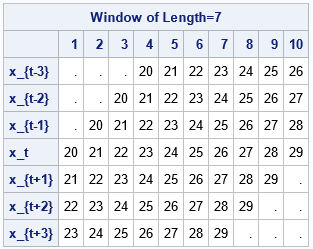The columns of the tables show the windows for the sequence of size n=10. The windows have radius k=3 (or length 2*k+1=7), which means that the centered window at y[t]includes the points
y[t-3], y[t-2], y[t-1], y[t], y[t+1], y[t+2], and y[t+3].
Complete windows are available for t=4, 5, 6, and 7. Near the ends of the sequence (when t ≤ k or t > n-k), you must decide what data values to use in the windows. In this example, I padded the sequence with missing values. Thus, the windows about the first and last data points contain only four (not seven) data points. An alternative is to use Tukey's padding (recommended by Velleman and Hoaglin, 1981, p. 161), which pads the sequence on the left by repeating y and pads the sequence on the right by repeating y[n]. Tukey's padding is the default method, so you can get Tukey's padding by calling the function as follows:

`M = extractWindows(y, 3); /* Tukey padding: Use y to pad the left and y[n] to pad the right */`

### Apply a moving statistic to each window

Many statistics in the SAS/IML language operate on columns of a data matrix. For example, the MEAN function (which can also compute trimmed and Winsorized means) computes the mean of each column. Similarly, the MEDIAN function computes the median of each column. The following statements read the cow's temperature into a SAS/IML vector and calculates the matrix of windows of length 7, using Tukey padding. The program then computes the median and trimmed mean of each column. These rolling statistics are written to a SAS data set and plotted by using PROC SGPLOT:

```use Cows; read all var {Day y}; close;   k = 3; M = extractWindows(y, k); /* Tukey padding; each column is a window of length 2*k+1 = 7 */ median = median(M); /* compute the moving median */ mean1 = mean(M, "trim", 1); /* compute the moving trimmed mean */ create Cows2 var{"Day", "Y", "Median", "Mean1"}; append; close; QUIT;   title "Overlay Running Median and Trimmed Mean"; title2 "Window Length = 7"; proc sgplot data=Cows2; scatter x=Day y=y; series x=Day y=Median / legendlabel="Running Median(7)" lineattrs=(thickness=2 color=DarkGreen); series x=Day y=Mean1 / legendlabel="Running Trimmed Mean(7)" lineattrs=(thickness=2 color=DarkRed); xaxis grid; yaxis grid label="Chirps (Body Temperature)"; run;```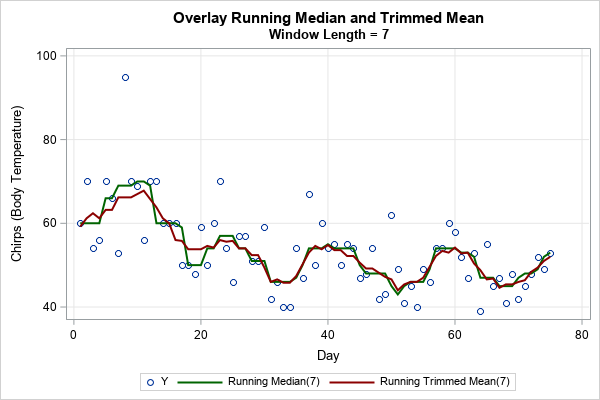The graph overlays two rolling robust statistics on the cow's temperature for each day. The rolling median is the same as is produced by PROC EXPAND except for the first and last k observations. (PROC EXPAND uses missing-value padding; the SAS/IML example uses Tukey padding.) The rolling trimmed mean is a different robust statistic, but both methods predict the same peaks for the cow's temperature.

### Summary

A rolling median is a robust statistic that can be used to smooth a time series that might have outliers. PROC EXPAND in SAS/ETS software supports the rolling median. However, you can also use SAS/IML to construct various rolling statistics.

This article shows how to use PROC IML to construct a matrix whose columns are moving windows. You can choose from two different ways to populate the windows at the beginning and end of the series. After you compute the matrix, you can apply many classical or robust statistics to the columns of the matrix. This gives you a lot of flexibility if you want to construct robust rolling statistics that are not supported by PROC EXPAND. This article shows an example of a trimmed mean. A future article will discuss using the MAD statistic to identify outliers in a time series.

The post The running median as a time series smoother appeared first on The DO Loop.You can standardize a numerical variable by subtracting a location parameter from each observation and then dividing by a scale parameter. Often, the parameters depend on the data that you are standardizing. For example, the most common way to standardize a variable is to subtract the sample mean and divide by the sample standard deviation. For a variable X, let c = mean(X) and s = std(X). Then one way to standardize X is to form the new variable
Y = (X - c) / s
which is a vector shorthand for the variable Y whose i_th observation is yi = (xi - c) / s.

Ironically, there are many ways to standardize a variable. You can use many different statistics to obtain estimates for c and s. You might want to do this is because the mean and standard deviation are not robust to the presence of outliers. For some data, a robust estimate of location and scale might be more useful. For example, you could choose the sample median as the location parameter (c) and a robust estimate of scale for the scale parameter (s). Robust estimates of scale include the interquartile range, the MAD statistic, and a trimmed standard deviation, to name a few.

When I want to standardize a set of variables, I use PROC STDIZE in SAS. It is a powerful tool that includes many built-in methods for standardizing variables. I previously wrote an article that shows several ways to use PROC STDIZE to standardize data.

You might not know that PROC STDIZE supports a way to perform your own custom standardizations. That is, PROC STDIZE supports nonstandard standardizations! Briefly, you can use any method to estimate the location and scale parameters for each variable and write those estimates to a SAS data set. You can then use the METHOD=IN(DataName) option on the PROC STDIZE statement to use those special estimates to standardize the variables. This article describes how to use the METHOD=IN option to perform a nonstandard standardization.

### The structure of the IN= data set

The IN= data set for PROC STDIZE must be in a specific format. Suppose you want to standardize three numerical variables: X1, X2, and X3. The IN= data set must contain variables that have the same names. The data set must also contain a character variable, _TYPE_. The rows specify parameters for each variable, and the _TYPE_ variable indicates which parameter is specified. For example, the following DATA step defines example data. A second data set specifies the location and scale parameters for each variable by using the format that is required by PROC STDIZE. In this example, the location and scale parameters for the X1 variable are 1 and 4, respectively. The parameters for the X2 variable are 2 and 5. The parameters for X3 are 3 and 6.

```/* specify the location and scale parameters for each var */ data Parms; length _TYPE_ \$14; input _TYPE_ /* required variables */ x1 x2 x3; /* names of variables that you are going to standardize */ datalines; LOCATION 1 2 3 SCALE 4 5 6 ;```

If you create a data set that contains variables X1, X2, and X3, you can use the parameters in the Parms data set to center and scale the variables, as follows:

```/* the raw data to be standardized */ data Have; input x1 x2 x3; datalines; 5 7 21 1 12 27 5 12 27 9 17 15 ;   /* use parameters in data set to standardize X1-X3 */ proc stdize data=Have out=Want method=in(Parms); var x1 x2 x3; run; proc print data=Want; run;```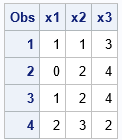The call to PROC STDIZE standardizes the variables by using the specified parameters. For example, the X2 variable is transformed according to the formula (X2 - 2)/5.

### Create an IN= data set from PROC MEANS

The previous example hard-coded the location and scale parameters for each variable. In practice, you often estimate the parameters by using statistics from procedures such as PROC SUMMARY, PROC MEANS, or PROC IML. This section shows how to create the METHOD=IN data set from the PROC MEANS output.

Suppose that you want the location parameter to be the sample means and the scale parameter to be the corrected sum of squares Σi (xi - m)2, where m is the sample mean. This combination of parameters is not one of the methods that are built into PROC STDIZE. However, you can use the METHOD=IN option to implement it.

First, use PROC MEANS to compute the means and corrected sum of squares (CSS). The statistics are in one long row. You can use a DATA step and arrays to reshape the data into the form required by PROC STDIZE:

```%let varNames = x1 x2 x3; %let locNames = c1-c3; %let scaleNames = s1-s3; proc means data=Have noprint; var &varNames; output out=Stats(drop=_TYPE_) mean=&locNames /* using mean to estimate location */ css=&scaleNames; /* using CSS for scale */ run;   /* the statistics are in one long row. Make one row for each statistic */ data Parms; length _TYPE_ \$14; array x[*] &varNames; /* new column names */ array loc[*] &locNames; /* column names for location parameter */ array scale[*] &scaleNames; /* column names for scale parameter */ set Stats; _TYPE_ = "LOCATION"; do i=1 to dim(loc); x[i] = loc[i]; end; output; _TYPE_ = "SCALE"; do i=1 to dim(scale); x[i] = scale[i]; end; output; keep _TYPE_ &varNames; run;   proc print data=Parms noobs; run;```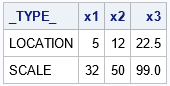For each variable, the location parameter is the sample mean. The scale parameter is the sample CSS. As before, you can use these values to standardize the raw data:

```/* use parameters in data set to standardize X1-X3 */ proc stdize data=Have out=Want2 method=in(Parms); var &varNames; run; proc print data=Want2; run;```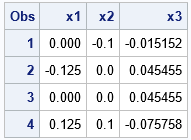### Summary

This article shows how to use PROC STDIZE in SAS to perform "nonstandard" standardizations. PROC STDIZE supports the METHOD=IN option, which enables you to specify a data set that contains the location and scale parameters. You can use SAS procedures (such as PROC MEANS) to compute and statistics you want. By writing the statistics to a data set and adding a _TYPE_ variable, you can standardize the variables by using any estimate of location and scale.

The post Nonstandard ways to standardize variables appeared first on The DO Loop.A previous article discusses the definition of the Hoeffding D statistic and how to compute it in SAS. The letter D stands for "dependence." Unlike the Pearson correlation, which measures linear relationships, the Hoeffding D statistic tests whether two random variables are independent. Dependent variables have a Hoeffding D statistic that is greater than 0. In this way, the Hoeffding D statistic is similar to the distance correlation, which is another statistic that can assess dependence versus independence.

This article shows a series of examples where Hoeffding's D is compared with the Pearson correlation. You can use these examples to build intuition about how the D statistic performs on real and simulated data.

### Hoeffding's D and duplicate values

As a reminder, Hoeffding's D statistic is affected by duplicate values in the data. If a vector has duplicate values, the Hoeffding association of the vector with itself will be less than 1. A vector that has many duplicate values (few distinct values) has an association with itself that might be much less than 1.

For many examples, the Hoeffding D association between two variables is between 0 and 1. However, occasionally you might see a negative value for Hoeffding's D, especially if there are many duplicate values in the data.

### Pearson correlation versus Hoeffding's D on real data

Let's compare the Pearson correlation and the Hoeffding D statistic on some real data. The following call to PROC SGSCATTER creates bivariate scatter plots for five variables in the Sashelp.Cars data set:

```%let VarList = MSRP Invoice EngineSize Horsepower MPG_City MPG_Highway; proc sgscatter data=sashelp.cars; matrix &VarList; run;```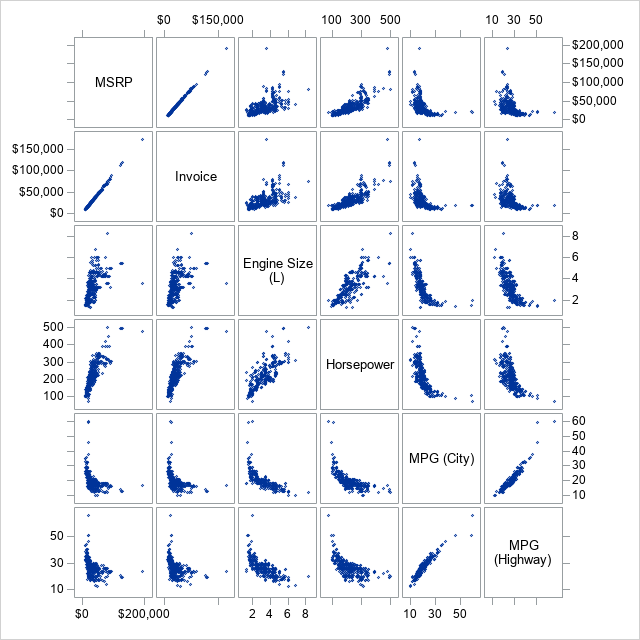The graph shows relationships between pairs of variables. Some pairs (MSRP and Invoice) are highly linearly related. Other pairs (MPG_City versus other variables) appear to be related in a nonlinear manner. Some pairs are positively correlated whereas others are negatively correlated.

Let's use PROC CORR in SAS to compare the matrix of Pearson correlations and the matrix of Hoeffding's D statistics for these pairs of variables. The NOMISS option excludes any observations that have a missing value in any of the specified variables:

```proc corr data=Sashelp.cars PEARSON HOEFFDING noprob nomiss nosimple; label EngineSize= MPG_City= MPG_Highway=; /* suppress labels */ var &VarList; run;```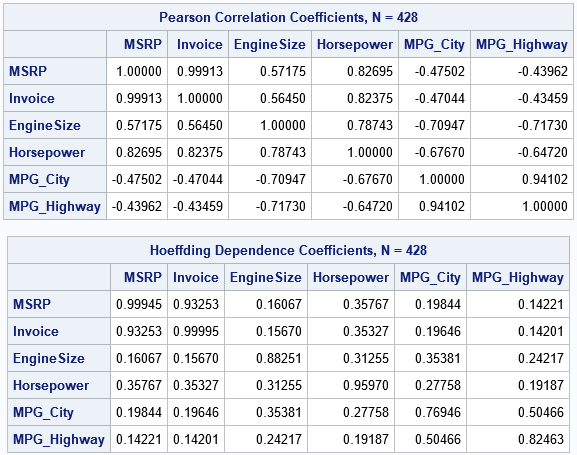There are a few noteworthy differences between the tables:

• Diagonal elements: For these variables, there are 428 complete cases. The Invoice variable has 425 unique values (only three duplicates) whereas the MPG_City and MPG_Highway variables have only 28 and 33 unique values, respectively. Accordingly, the diagonal elements are closest to 1 for the variables (such as MSRP and Invoice) that have few duplicate values and are smaller for variables that have many duplicate values.
• Negative correlations: A nice feature of the Pearson correlation is that it reveals positive and negative relationships. Notice the negative correlations between MPG_City and other variables. In contrast, the Hoeffding association assesses dependence/independence. The association between MPG_City and the other variables is small, but the table of Hoeffding statistics does not give information about the direction of the association.
• Magnitudes: The off-diagonal Hoeffding D statistics are mostly small values between 0.15 and 0.35. In contrast, the same cells for the Pearson correlation are between -0.71 and 0.83. As shown in a subsequent section, the Hoeffding statistic has a narrower range than the Pearson correlation does.

### Association of exact relationships

A classic example in probability theory shows that correlation and dependence are different concepts. If X is a random variable and Y=X2, then X and Y are not independent, even though their Pearson correlation is 0. The following example shows that the Hoeffding statistic is nonzero, which indicates dependence between X and Y:

```data Example; do x = -1 to 1 by 0.05; /* X is in [-1, 1] */ yLinear = 3*x + 2; /* Y1 = 3*X + 2 */ yQuad = x**2 + 1; /* Y2 = X**2 + 1 */ output; end; run;   proc corr data=Example PEARSON HOEFFDING nosimple noprob; var x YLinear yQuad; run;```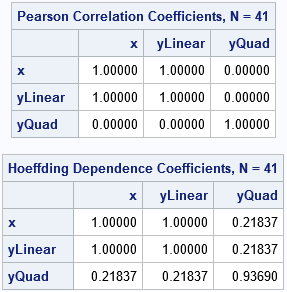Both statistics (Pearson correlation and Hoeffding's D) have the value 1 for the linear dependence between X and YLinear. The Pearson correlation between X and YQuad is 0, whereas the Hoeffding D statistic is nonzero. This agrees with theory: the two random variables are dependent, but their Pearson correlation is 0.

### Statistics for correlated bivariate normal data

A previous article about distance correlation shows how to simulate data from a bivariate normal distribution with a specified correlation. Let's repeat that numerical experiment, but this time compare the Pearson correlation and the Hoeffding D statistic. To eliminate some of the random variation in the statistics, let's repeat the experiment 10 times and plot the Monte Carlo average of each experiment. That is, the following SAS/IML program simulates bivariate normal data for several choices of the Pearson correlation. For each simulated data set, the program computes both the Pearson correlation and Hoeffding's D statistic. This is repeated 10 times, and the average of the statistics is plotted:

```proc iml; call randseed(54321);   /* helper functions */ start PearsonCorr(x,y); return( corr(x||y)[1,2] ); finish; start HoeffCorr(x,y); return( corr(x||y, "Hoeffding")[1,2] ); finish;   /* grid of correlations */ rho = {-0.99 -0.975 -0.95} || do(-0.9, 0.9, 0.1) || {0.95 0.975 0.99}; N = 500; /* sample size */ mu = {0 0}; Sigma = I(2); /* parameters for bivariate normal distrib */ PCor = j(1, ncol(rho), .); /* allocate vectors for results */ HoeffD = j(1, ncol(rho), .);   /* generate BivariateNormal(rho) data */ numSamples = 10; /* how many random samples in each study? */ do i = 1 to ncol(rho); /* for each rho, simulate bivariate normal data */ Sigma[1,2] = rho[i]; Sigma[2,1] = rho[i]; /* population covariance */ meanP=0; meanHoeff=0; /* Monte Carlo average in the study */ do k = 1 to numSamples; Z = RandNormal(N, mu, Sigma); /* simulate bivariate normal sample */ meanP = meanP + PearsonCorr(Z[,1], Z[,2]); /* Pearson correlation */ meanHoeff = meanHoeff + HoeffCorr(Z[,1], Z[,2]); /* Hoeffding D */ end; PCor[i] = MeanP /numSamples; /* MC mean of Pearson correlation */ HoeffD[i] = meanHoeff / numSamples; /* MC mean of Hoeffding D */ end;   create MVNorm var {"rho" "PCor" "HoeffD"}; append; close; QUIT;   title "Average Correlations/Associations of Bivariate Normal Data"; title2 "N = 500"; proc sgplot data=MVNorm; label rho="Pearson Correlation of Population" PCor="Pearson Correlation" HoeffD="Hoeffding's D"; series x=rho y=PCor / markers name="P"; series x=rho y=HoeffD / markers name="D"; lineparm x=0 y=0 slope=1; yaxis grid label="Association or Correlation"; xaxis grid; keylegend "P" "D"; run;```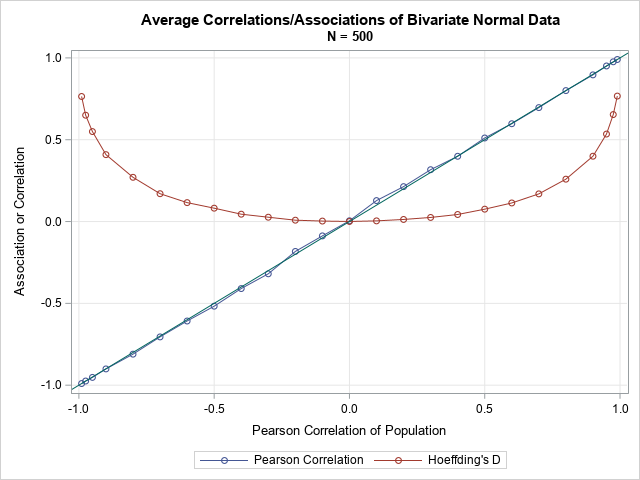For these bivariate normal samples (of size 500), the Monte Carlo means for the Pearson correlations are very close to the diagonal line, which represented the expected value of the correlation. For the same data, the Hoeffding D statistics are different. For correlations in [-0.3, 0.3], the mean of the D statistic is positive and is very close to 0. Nevertheless, the p-values for the Hoeffding test of independence account for the shape of the curve. For bivariate normal data that has a small correlation (say, 0.1), the Pearson test for zero correlation and the Hoeffding test for independence often both accept or both reject their null hypotheses.

### Summary

Hoeffding's D statistic provides a test for independence, which is different than a test for correlation. In SAS, you can compute the Hoeffding D statistic by using the HOEFFDING option on the PROC CORR statement. You can also compute it in SAS/IML by using the CORR function. Hoeffding's D statistic can detect nonlinear dependencies between variables. It does not, however, indicate the direction of dependence.

I doubt Hoeffding's D statistic will ever be as popular as Pearson's correlation, but you can use it to detect nonlinear dependencies between pairs of variables.

The post Examples of using the Hoeffding D statistic appeared first on The DO Loop.